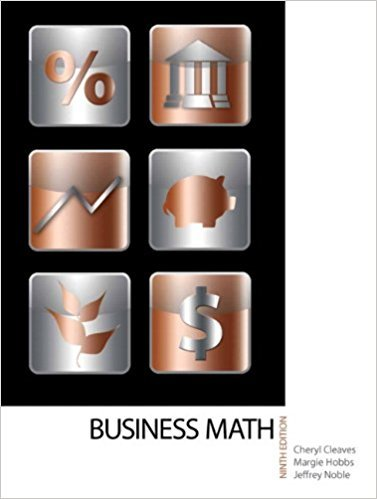×
×

# Solutions for Chapter 20-3: INCOME TAXES## Full solutions for Business Math, | 9th Edition

ISBN: 9780135108178Solutions for Chapter 20-3: INCOME TAXES

Solutions for Chapter 20-3
4 5 0 427 Reviews
15
1
##### ISBN: 9780135108178

This expansive textbook survival guide covers the following chapters and their solutions. This textbook survival guide was created for the textbook: Business Math, , edition: 9. Since 20 problems in chapter 20-3: INCOME TAXES have been answered, more than 17478 students have viewed full step-by-step solutions from this chapter. Business Math, was written by and is associated to the ISBN: 9780135108178. Chapter 20-3: INCOME TAXES includes 20 full step-by-step solutions.

Key Math Terms and definitions covered in this textbook
• Block matrix.

A matrix can be partitioned into matrix blocks, by cuts between rows and/or between columns. Block multiplication ofAB is allowed if the block shapes permit.

• Circulant matrix C.

Constant diagonals wrap around as in cyclic shift S. Every circulant is Col + CIS + ... + Cn_lSn - l . Cx = convolution c * x. Eigenvectors in F.

• Cofactor Cij.

Remove row i and column j; multiply the determinant by (-I)i + j •

• Cramer's Rule for Ax = b.

B j has b replacing column j of A; x j = det B j I det A

• Echelon matrix U.

The first nonzero entry (the pivot) in each row comes in a later column than the pivot in the previous row. All zero rows come last.

• Elimination.

A sequence of row operations that reduces A to an upper triangular U or to the reduced form R = rref(A). Then A = LU with multipliers eO in L, or P A = L U with row exchanges in P, or E A = R with an invertible E.

• Fibonacci numbers

0,1,1,2,3,5, ... satisfy Fn = Fn-l + Fn- 2 = (A7 -A~)I()q -A2). Growth rate Al = (1 + .J5) 12 is the largest eigenvalue of the Fibonacci matrix [ } A].

• Four Fundamental Subspaces C (A), N (A), C (AT), N (AT).

Use AT for complex A.

• Gram-Schmidt orthogonalization A = QR.

Independent columns in A, orthonormal columns in Q. Each column q j of Q is a combination of the first j columns of A (and conversely, so R is upper triangular). Convention: diag(R) > o.

• Nilpotent matrix N.

Some power of N is the zero matrix, N k = o. The only eigenvalue is A = 0 (repeated n times). Examples: triangular matrices with zero diagonal.

• Normal equation AT Ax = ATb.

Gives the least squares solution to Ax = b if A has full rank n (independent columns). The equation says that (columns of A)·(b - Ax) = o.

• Orthogonal matrix Q.

Square matrix with orthonormal columns, so QT = Q-l. Preserves length and angles, IIQxll = IIxll and (QX)T(Qy) = xTy. AlllAI = 1, with orthogonal eigenvectors. Examples: Rotation, reflection, permutation.

• Particular solution x p.

Any solution to Ax = b; often x p has free variables = o.

• Pivot columns of A.

Columns that contain pivots after row reduction. These are not combinations of earlier columns. The pivot columns are a basis for the column space.

• Rank r (A)

= number of pivots = dimension of column space = dimension of row space.

• Row space C (AT) = all combinations of rows of A.

Column vectors by convention.

• Saddle point of I(x}, ... ,xn ).

A point where the first derivatives of I are zero and the second derivative matrix (a2 II aXi ax j = Hessian matrix) is indefinite.

• Symmetric factorizations A = LDLT and A = QAQT.

Signs in A = signs in D.

• Trace of A

= sum of diagonal entries = sum of eigenvalues of A. Tr AB = Tr BA.

• Vandermonde matrix V.

V c = b gives coefficients of p(x) = Co + ... + Cn_IXn- 1 with P(Xi) = bi. Vij = (Xi)j-I and det V = product of (Xk - Xi) for k > i.

×